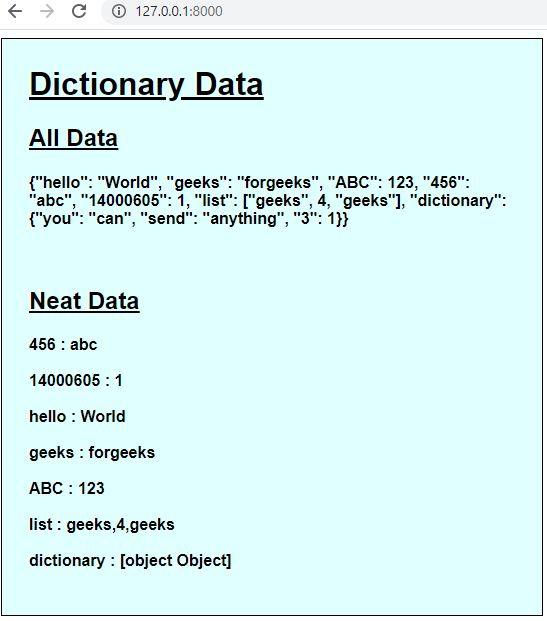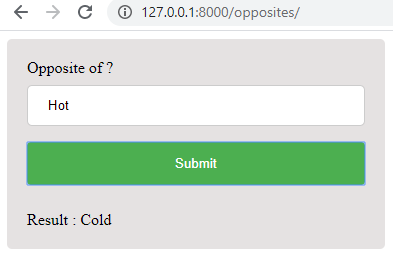# How to pass data to javascript in Django Framework ?

Django is a python framework for web development which works on jinja2 templating engine. When data is rendered along with the template after passing through views.py, that data becomes static on the html file along which it was rendered. As django is a backend framework, hence to use the power of python to use that data dynamically requests need to be generated. These requests can be type GET, POST, AJAX etc. But without making any call to the backend the only way to use that data dynamically is to pass it to JavaScript. Often passing a few values to JavaScript does the trick. But sometimes the entire dictionary of data needs to passed. This can be done using JSON and django template tags.

To check more about django framework in python visit – Django Tutorial.

In ‘views.py’ file –

 `from` `django.shortcuts ``import` `render ` `from` `json ``import` `dumps ` ` `  ` `  `def` `send_dictionary(request): ` `    ``# create data dictionary ` `    ``dataDictionary ``=` `{ ` `        ``'hello'``: ``'World'``, ` `        ``'geeks'``: ``'forgeeks'``, ` `        ``'ABC'``: ``123``, ` `        ``456``: ``'abc'``, ` `        ``14000605``: ``1``, ` `        ``'list'``: [``'geeks'``, ``4``, ``'geeks'``], ` `        ``'dictionary'``: {``'you'``: ``'can'``, ``'send'``: ``'anything'``, ``3``: ``1``} ` `    ``} ` `    ``# dump data ` `    ``dataJSON ``=` `dumps(dataDictionary) ` `    ``return` `render(request, ``'main / landing.html'``, {``'data'``: dataJSON}) `

In ‘landing.html’ file –

 ` ` `<``body``> ` `    ``<``div` `style="width: 40%; ` `    ``border: 1px solid black; ` `    ``background-color: lightcyan; ` `    ``font-family: Helvetica, sans-serif;"> ` `        ``<``div` `style``=``"margin: 5%;"``> ` `            ``<``h1``> ` `                ``<``u``>Dictionary Data ` `            `` ` `            ``<``h2``> ` `                ``<``u``>All Data ` `            `` ` `            ``<``h4` `id``=``'alldata'``><``br``> ` `            ``<``h2``> ` `                ``<``u``>Neat Data ` `            `` ` `            ``<``h4` `id``=``'neatdata'``> ` `        `` ` `    `` ` ` ` ` ` `<``script``> ` `    ``var data = JSON.parse("{{data|escapejs}}"); ` `    ``var dataNode = document.getElementById('alldata'); ` `    ``dataNode.innerHTML+="{{data|escapejs}}"; ` `    ``dataNode = document.getElementById('neatdata'); ` `    ``for(var x in data){ ` `        ``dataNode.innerHTML+=x+' : '+data[x]+'<``br``><``br``>'; ` `    ``} ` ``

Output:Now, the value of “dictionary” is not the same as we entered in python. This is because JavaScript considers dictionaries as separate objects so to view the data of those objects we have to specify separately in JavaScript.

## Use Case –

Let’s create an opposite finder website using Django. We will feed the opposite relation between words to Python and send it to JavaScript to determine the output based on user’s input.

In ‘views.py’ file –

 `from` `django.shortcuts ``import` `render ` `from` `json ``import` `dumps ` ` `  ` `  `def` `opposites(request): ` `    ``# create data dictionary ` `    ``data ``=` `[ ` `        ``[``"Laugh"``, ``"Cry"``], ` `        ``[``"Even"``, ``"Odd"``], ` `        ``[``"Hot"``, ``"Cold"``], ` `        ``[``"Light"``, ``"Dark"``], ` `        ``[``"Opposite"``, ``"Same"``], ` `        ``[``"Far"``, ``"Near"``], ` `        ``[``"Give"``, ``"Take"``], ` `        ``[``"Night"``, ``"Day"``], ` `        ``[``"Import"``, ``"Export"``], ` `        ``[``"Hard"``, ``"Easy"``], ` `        ``[``"Never"``, ``"Always"``], ` `        ``[``"Late"``, ``"Early"``], ` `        ``[``"Less"``, ``"More"``], ` `        ``[``"Male"``, ``"Female"``], ` `        ``[``"Happiness"``, ``"Sadness"``], ` `        ``[``"Fast"``, ``"Slow"``], ` `        ``[``"Old"``, ``"Young"``], ` `        ``[``"Boy"``, ``"Girl"``], ` `        ``[``"Up"``, ``"Down"``], ` `        ``[``"Left"``, ``"Right"``], ` `        ``[``"Rich"``, ``"Poor"``], ` `        ``[``"Love"``, ``"Hate"``], ` `        ``[``"Inside"``, ``"Outside"``], ` `        ``[``"Bad"``, ``"Good"``], ` `        ``[``"Short"``, ``"Tall"``], ` `    ``] ` `    ``data ``=` `dumps(data) ` `    ``return` `render(request, ``"main/opposites.html"``, {``"data"``: data}) `

In ‘opposites.html’ file –

 ` ` `<``html``> ` `<``body``> ` `<``div``> ` `  ``Opposite of ? ` `  ``<``input` `type``=``"text"` `id``=``"input"` `placeholder``=``"Input"``> ` `  ``<``input` `type``=``"submit"` `id``=``'opp'` `value``=``"Submit"` `onclick``=``"opposite()"``> ` `  ``<``br``><``br``>Result : <``h` `id``=``'result'``> ` ` ` ` ` ` ` `<``script``> ` `  ``function opposite(){ ` `    ``var data = JSON.parse("{{data|escapejs}}"); ` `    ``var input = document.getElementById("input").value; ` `    ``var result = document.getElementById("result") ` `    ``var flag = 1; ` `    ``for(var x in data){ ` `      ``if(input.toLowerCase()==data[x].toLowerCase()){ ` `        ``result.innerHTML = data[x]; ` `        ``flag=0; ` `      ``} ` `      ``else if(input.toLowerCase()==data[x].toLowerCase()){ ` `        ``result.innerHTML = data[x]; ` `        ``flag=0; ` `      ``} ` `    ``} ` `    ``if(flag){ ` `      ``result.innerHTML = "No results found" ` `    ``} ` `  ``} ` ``

Output:Attention geek! Strengthen your foundations with the Python Programming Foundation Course and learn the basics.

To begin with, your interview preparations Enhance your Data Structures concepts with the Python DS Course.

My Personal Notes arrow_drop_upPython Developer | Machine Learning Enthusiast | WebDevDjango

If you like GeeksforGeeks and would like to contribute, you can also write an article using contribute.geeksforgeeks.org or mail your article to contribute@geeksforgeeks.org. See your article appearing on the GeeksforGeeks main page and help other Geeks.

Please Improve this article if you find anything incorrect by clicking on the "Improve Article" button below.

Article Tags :

1

Please write to us at contribute@geeksforgeeks.org to report any issue with the above content.